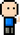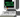# Josh Meets Computer# Social Network Prediction Problem

April 17, 2018

Given a fixed network, can we predict how the network is going to look in the future? This certain problem has a lot of applications in Social Networks; given a group of friends, can you build an effective recommender system to suggest new connections?

## Network Measurements

### Common Neighbors

common_neighbors = N1neighbors ∪ N2neighbors

``````import networkx as nx

def common_neighbors(G, n1, n2):
return len(set(G.neighbors(n1)) & set(G.neighbors(n2)))

G = nx.karate_club_graph()

# get number of common neighbors for all nodes that are not connected
c = [(e, e, common_neighbors(G, e, e)) for e in nx.non_edges(G)]

node1 node2 Common Neighbors
0 0 32 3
1 0 33 4
2 0 9 1
3 0 14 0
4 0 15 0

### Jaccard Coefficient

Similar to Common neighbors; denotes the number of common neighbors normalized by total number of neighbors. A Jaccard Coefficient of 1 indicates that two unconnected nodes share all the same neighbors. An example of this would be a triadic closure — node A and B are both only connected to C, but not connected themselves. They both share C, and the total number of neighbors is just C. This would indicate a high probability of the two nodes connecting.

``````import pandas as pd
def total_neighbors(G, n1, n2):
return len(set(G.neighbors(n1)) | set(G.neighbors(n2)))

def jaccard_coefficient(G):
for n in nx.non_edges(G):
jaccard = common_neighbors(G, n, n) / total_neighbors(G, n, n)
yield (n, n, jaccard)

pd.DataFrame([i for i in jaccard_coefficient(G)], columns=['node1', 'node2', 'Jaccard Coeff.']).head()``````
node1 node2 Jaccard Coeff.
0 0 32 0.120000
1 0 33 0.137931
2 0 9 0.058824
3 0 14 0.000000
4 0 15 0.000000

### Resource Allocation Index

describes the fraction of a ‘resource’ that one node can send to another through their respective neighbors. It is calculated by looked at each of the common neighbor nodes, and summing the inverse of degree for each of these nodes. The intution is that if a lot of the common neighbor nodes have low degrees, then there is a high likelihood that information being sent from the first node to the second (through the common neighbors) will reach the second node. If their common neighbors have a ton of other connections (higher degree), this will result in a lower Resource Allocation Index. At the same time, since the index sums over all common neighbors, two nodes with a lot of common neighbors will have more opportunities to send information to each other, denoting a higher Resource Allocation Index as well.

``````import matplotlib.pyplot as plt

def resource_allocation_index(G):
for n in nx.non_edges(G):
common_neighbors = set(G.neighbors(n)) & set(G.neighbors(n))
index = 0

for c_n in common_neighbors:
index += 1 / len(G.neighbors(c_n))
yield (n, n, index)

df = pd.DataFrame([i for i in resource_allocation_index(G)], columns=['Node 1', 'Node 2', 'RAI'])
Node 1 Node 2 RAI
0 0 32 0.466667
1 0 33 0.900000
2 0 9 0.100000
3 0 14 0.000000
4 0 15 0.000000

Similar to Resource Allocation Index, but divides by the log of the degree for each common neighbor instead of just the degree

``````import math

for n in nx.non_edges(G):
common_neighbors = set(G.neighbors(n)) & set(G.neighbors(n))
index = 0
for a_n in common_neighbors:
index += 1 / math.log(len(G.neighbors(a_n)))
yield (n, n, index)

df = pd.DataFrame([i for i in adamic_adar_index(G)], columns=['Node 1', 'Node 2', 'AAI'])
Node 1 Node 2 AAI
0 0 32 1.613740
1 0 33 2.711020
2 0 9 0.434294
3 0 14 0.000000
4 0 15 0.000000

### Preferential Attachment Score

Intuition is that if two nodes have a very high degree, it’s very likely that they’ll be connected in the future. Two popular nodes (they are connected to a lot of other nodes) stand a very good chance at becoming connected (aware) of each other.

``````def preferential_attachment_score(G):
for n in nx.non_edges(G):
yield (n, n, len(G.neighbors(n)) * len(G.neighbors(n)))

df = pd.DataFrame([i for i in preferential_attachment_score(G)], columns=['Node 1', 'Node 2', 'PAS'])
Node 1 Node 2 PAS
0 0 32 192
1 0 33 272
2 0 9 32
3 0 14 32
4 0 15 32

## Community Structure of Network

Pairs of nodes who belong to the same community and have many common neighbors have a high likelihood of forming an edge. This measures the number of common neighbors between two nodes, but gives a bonus to this measurement if the two nodes belong to the same community.

### Common Neighbor Soundarajan-Hopcroft Score

This score indicates the count of common neighbors between two nodes, summed together with the count of common neighbors that belong to the same community as the two nodes.

``````nx.set_node_attributes(G, 'community', 1)

def community(G, n):
com = nx.get_node_attributes(G, 'community')
return com[n]

def soundarajan_hopcroft_score(G):
for n in nx.non_edges(G):
common_neighbors = set(G.neighbors(n)) & set(G.neighbors(n))
score = 0
for c_n in common_neighbors:
score += 1 + (lambda n, c: 1 if community(G, n) == community(G, n) == community(G, c) else 0)(n, c_n)

yield (n, n, score)

df = pd.DataFrame([i for i in soundarajan_hopcroft_score(G)], columns=['Node 1', 'Node 2', 'SHC'])
Node 1 Node 2 SHC
0 0 32 6
1 0 33 8
2 0 9 2
3 0 14 0
4 0 15 0

### Resource Allocation Soundarajan-Hopcroft Score

Similar to Resource Aloocation Score, counting the number of degrees each common neighbor has between two nodes. However there is an additional filter criteria where it only counts these instances when the common neighbor is in the same community as the two nodes.

``````def community(G, n):
com = nx.get_node_attributes(G, 'community')
return com[n]

def community_degrees(G, n, c):
"""
A function to calculate the individual Resource Allocation Soundarajan Hopcroft
Score for each common neighbor node.
args:
G (graph): the graph
n (tuple): two non connected nodes
c (int): a single node that is a connected neighbor of the two non connected nodes

returns:
score: the resource allocation score of this common node
"""

if community(G, c) == community(G, n) == community(G, n):
return 1 / len(G.neighbors(c))
else:
return 0

def resource_allocation_soundarajan_hopcroft(G):
for n in nx.non_edges(G):
common_neighbors = set(G.neighbors(n)) & set(G.neighbors(n))
score = 0
for c_n in common_neighbors:
score += community_degrees(G, n, c_n)
yield (n, n, score)

df = pd.DataFrame([i for i in resource_allocation_soundarajan_hopcroft(G)], columns=['Node 1', 'Node 2', 'RA-SHS'])